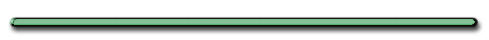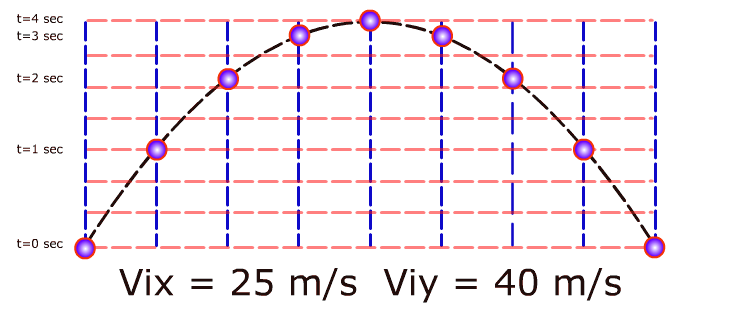Projectile Motion - VolleyballProjectile Motion

showmethphysics.comProjectile

Where Vix = 25 m/s

Viy = 40 m/s

 t (sec) Vx (m/s) Vy (m/s) 0 25 40 1 25 30 2 25 20 3 25 10 4 25 0 (peak) 5 25 -10 6 25 -20 7 25 -30 8 25 -40

4. Word Problems

www.sihunt.co.uk Russian long jumper Tayana Lebedeva performs a
double hitch action in the 2007 world finals in Osaka Japan.

 Ex) An athlete doing a running jump leaves ground at an angle of 25.° and a velocity of 10. m/s. (a) What is the initial vertical component (Viy) of the athlete's velocity?

Ex)  An athlete doing a running jump leaves ground at an angle of 25.° and a velocity of 10. m/s.

(a) What is the initial vertical component (Viy) of the athlete's velocity?Viy = VisinӨ

= (10. m/s)sin25.°

= 4.2 m/s up

(b) How long does it take for
the athlete to reach her maximum height?

(b) How long does it take for
the athlete to reach her
maximum height?

 X Y t = ? Viy = 4.2 m/s (from before) t  =  ? Vfy= 0 (peak) ay = -9.8 m/s2

Vf = Vi + at

0 =4.2 m/s+(-9.8 m/s2)t

(subtract 4.2 m/s from both sides)

- 4.2 m/s = (-9.8 m/s2)t

(- 4.2 m/s)/(-9.8 m/s2) = t

t = .43 secs
to reach peak

(c) How long did it take for the athlete to complete the entire jump?

(c) How long did it take the athlete to complete her entire jump?

On level ground

 Total time = double peak time

Entire Jump:

Total Time = .86 seconds(c) How far did she jump?

dx = ?

(c) How far did she jump?

dx = ?

t = .86 sec

dx = Vxt

(Must find Vx first)

Vx = VcosӨ

Vx = (10. m/s)cos25.°

Vx = 9.1 m/s

dx = 9.1 m/s(.86 sec)

dx = 7.8 m